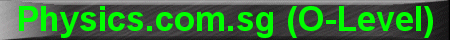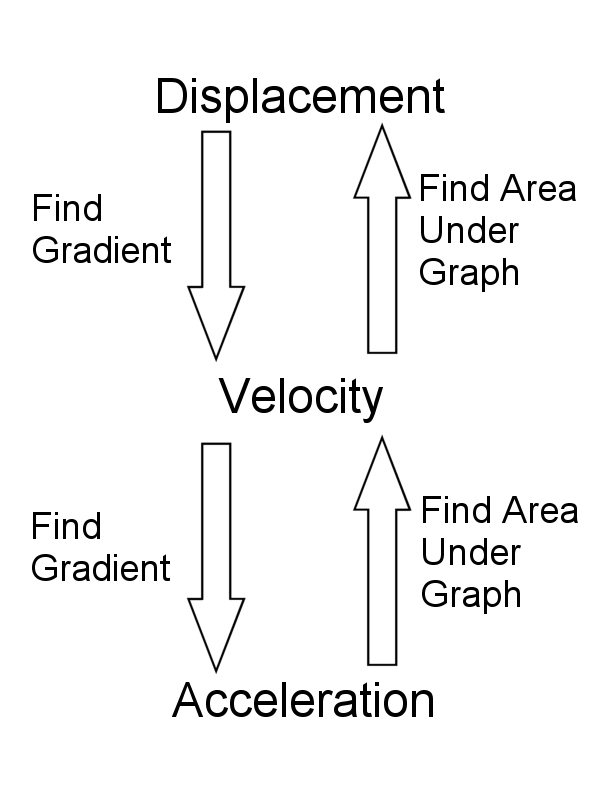(New tips are continually added to these pages.  Check back in a few months' time for more)

TOPIC 2:     Speed, Velocity and Acceleration

Tip 1:

Distance and Speed are scalar quantities, ie. they have only magnitude but no direction.

Displacement, Velocity and Acceleration are vector quantities, ie. they have both magnitude and direction.

Displacement, Velocity and Acceleration's relationship is like that between a Grandfather, Father and Son.

Displacement is the area under the velocity-time graph (Grandfather is the father of Father).

Velocity is the area under the acceleration-time graph (Father is the the father of Son).

Velocity is the rate of change of displacement, or gradient of the displacement-time graph (Father is the son of Grandfather).

Acceleration is the rate of change of velocity, or gradient of the velocity-time graph (Son is the son of Father).